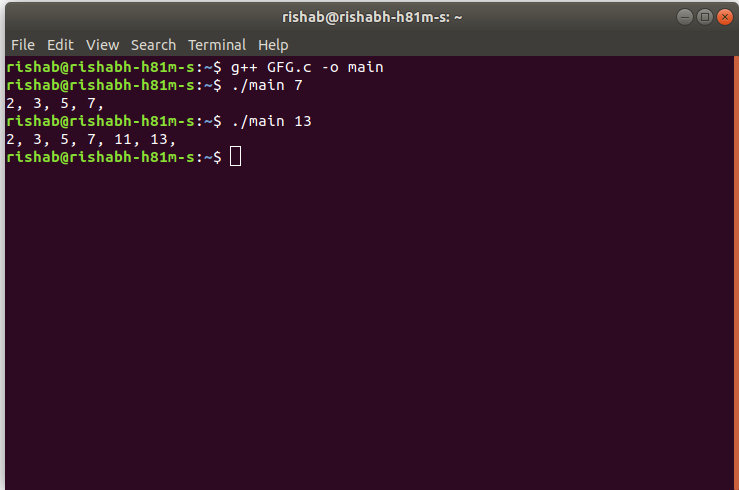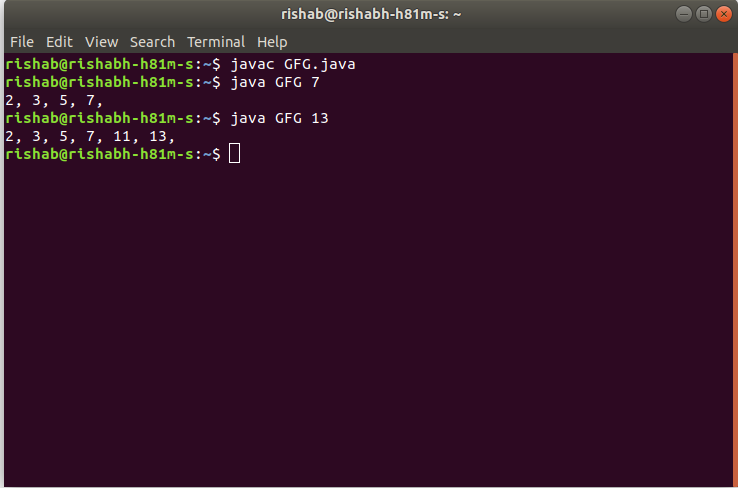GeeksforGeeks App
Open AppBrowser
Continue

# TCS Coding Practice Question | Prime Numbers upto N

Given a number N, the task is to find the Prime Numbers from 1 to N using Command Line Arguments.

Examples:

Input: N = 7
Output: 2, 3, 5, 7

Input: N = 13
Output: 2, 3, 5, 7, 11, 13

Approach:

• Since the number is entered as Command line Argument, there is no need for a dedicated input line
• Extract the input number from the command line argument
• This extracted number will be in String type.
• Convert this number into integer type and store it in a variable, say N
• Now loop through the numbers from 2 to N, using a variable say i, to check if they are prime or not. This is done as follows:
In each iteration,
• Check if any numbers from 2 to (i/2+1) divide i completely (i.e. if it is a factor of i).
• If yes, then i is not a prime number. Hence check for next number
• If no, then i is a prime number. Hence print the value of i and check for next number
• After the loop has ended, the prime numbers from 1 to N are printed on the screen.

Note: Please note that 1 is not checked in this scenarios because 1 is neither prime nor composite.

Program:

## C

 `// C program to find``// the Prime Numbers from 1 to N``// using command line arguments`` ` `#include `` ` `#include /* atoi */`` ` `// Function to check if x is prime``int` `isPrime(``int` `x)``{``    ``int` `i;`` ` `    ``// Loop to check if x has any factor``    ``// other than 1 and x itself``    ``for` `(i = 2; i < x / 2 + 1; i++) {``        ``if` `(x % i == 0) {``            ``// Since i is a factor of x``            ``// x is not prime``            ``return` `0;``        ``}``    ``}`` ` `    ``// x is prime``    ``return` `1;``}`` ` `// Function to find prime numbers up to n``void` `findPrimes(``int` `n)``{``    ``int` `i;`` ` `    ``// Loop from 2 to n``    ``// to find all prime numbers in between``    ``for` `(i = 2; i <= n; i++) {`` ` `        ``// Check if i is prime``        ``// If yes then print it``        ``// else continue to next number``        ``if` `(isPrime(i) == 1)``            ``printf``(``"%d, "``, i);``    ``}`` ` `    ``printf``(``"\n"``);``}`` ` `// Driver code``int` `main(``int` `argc, ``char``* argv[])``{`` ` `    ``int` `n;`` ` `    ``// Check if the length of args array is 1``    ``if` `(argc == 1)``        ``printf``(``"No command line arguments found.\n"``);``    ``else` `{`` ` `        ``// Get the command line argument and``        ``// Convert it from string type to integer type``        ``// using function "atoi( argument)"``        ``n = ``atoi``(argv);`` ` `        ``// Find all prime numbers upto n``        ``findPrimes(n);``    ``}``    ``return` `0;``}`

## Java

 `// Java program to find``// the Prime Numbers from 1 to N``// using command line arguments`` ` `class` `GFG {`` ` `    ``// Function to check if x is prime``    ``public` `static` `int` `isPrime(``int` `x)``    ``{``        ``int` `i;`` ` `        ``// Loop to check if x has any factor``        ``// other than 1 and x itself``        ``for` `(i = ``2``; i < x / ``2` `+ ``1``; i++) {``            ``if` `(x % i == ``0``) {``                ``// Since i is a factor of x``                ``// x is not prime``                ``return` `0``;``            ``}``        ``}`` ` `        ``// x is prime``        ``return` `1``;``    ``}`` ` `    ``// Function to find prime numbers up to n``    ``public` `static` `void` `findPrimes(``int` `n)``    ``{``        ``int` `i;`` ` `        ``// Loop from 2 to n``        ``// to find all prime numbers in between``        ``for` `(i = ``2``; i <= n; i++) {`` ` `            ``// Check if i is prime``            ``// If yes then print it``            ``// else continue to next number``            ``if` `(isPrime(i) == ``1``)``                ``System.out.print(i + ``", "``);``        ``}`` ` `        ``System.out.println();``    ``}`` ` `    ``// Driver code``    ``public` `static` `void` `main(String[] args)``    ``{`` ` `        ``// Check if length of args array is``        ``// greater than 0``        ``if` `(args.length > ``0``) {`` ` `            ``// Get the command line argument and``            ``// Convert it from string type to integer type``            ``int` `n = Integer.parseInt(args[``0``]);`` ` `            ``// Find all prime numbers upto n``            ``findPrimes(n);``        ``}``        ``else``            ``System.out.println(``"No command line "``                               ``+ ``"arguments found."``);``    ``}``}`

Output:

• In C:• In Java:Time Complexity: O(N*N)
Auxiliary Space: O(1)

My Personal Notes arrow_drop_up